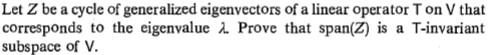# Let Z be a cycle of generalized eigenvectors of a linear operator T on V thatcorresponds to the eigenvalue 2 Prove that span(Z) is a T-invariantsubspace of V.

Question
1 viewshelp_outlineImage TranscriptioncloseLet Z be a cycle of generalized eigenvectors of a linear operator T on V that corresponds to the eigenvalue 2 Prove that span(Z) is a T-invariant subspace of V. fullscreen
check_circle

Step 1

Hi! This is an Advanced Math question. We are answering it now but kindly post such questions under Advanced Math now onward.

Here, we are given that Z is the cycle of generalized eigenvectors of a linear operator T on V that corresponds to the eigenvalue λ.

Let T be a linear operator on a vector space V, and let λ be an eigenvalue of T. Suppose that

Z = { v1, v2, ... ,vn }

Or

if p is is the smallest positive integer such that (T- λI)pv = 0, Z = {(T- λI)p-1v, (T- λI)p-2v, ... , (T- λI)v, v},

cycles of generalized eigenvectors of T corresponding to λ.

Note:

The generalized eigenvectors in a chain are linearly independent.

...

### Want to see the full answer?

See Solution

#### Want to see this answer and more?

Solutions are written by subject experts who are available 24/7. Questions are typically answered within 1 hour.*

See Solution
*Response times may vary by subject and question.
Tagged in